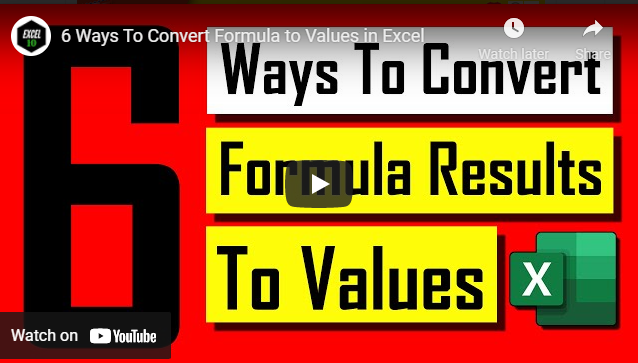# 6 ways to remove formulas and keep values in ExcelConverting formula results into value is crucial. Every now and then we need to remove formulas, keeping the results as values, and here you’ll learn 6 ways to do that.

In this Excel tutorial for beginners, you will find the 6 most efficient ways to replace a formula with its result. As a beginner, it's really important for you. As you go forward, every now and then you’ll need to use excel formulas and functions. Most of the functions require multiple references, and you will need to write the formula in an empty cell. Once you find the result, you might need to remove the reference cell, row, or column. This will be where you’ll get an error in your formula. To workaround this, you need to convert the formula into value. For example, you have your first name in column A and your last name in column B. Now you need to join the first name and last name in column C. You can use a function to do it like I showed in this tutorial. Now what if you need to delete column A and B? If you remove these two columns, your formula will show an error. Sometimes we might need to remove the entire formula while keeping the formula. These are the most common yet crucial scenarios that you are going to come across.

So we are going to solve this in six different ways. They are as follows.

Procedure 1: Here we are going to use a notepad or any other text editor to clear up the formula in Excel by keeping data. First, copy the cells where you have the formula and paste it into the notepad. Copy them again and paste them into the cell from where you copied them in the first place. This was my only way when I was a beginner.

Procedure 2: Start by coping the cells with the formula. Now click on the "Home" tab from the "Ribbon". Look for "Clipboard Group". Now click on the dropdown menu written below the "Paste Icon" and select "as value". This will remove the formula and keep only the value.

Procedure 3: All you need is a mouse for this one. Before pressing right click copy the cell with the formula. After that click on "As values". This will remove the formula and paste the formula result only.

Procedure 4: Here comes the shortcut to remove formula keeping the values in Excel. But before pressing the shortcut, you need to copy the formula cell and then press Alt + E + S + V. Now click OK and you're done!

Procedure 5: Select and copy the cell with the formula. Now press Shift + F10. Once you see the context menu, press V. This will also remove the formula and keep values only.

Procedure 6: This is going to be the best way to change the formula result into a value. Hover your mouse over the cell gridline. You’ll see your mouse cursor change into a four-sided arrow. Now, by pressing the right mouse button, drag and place where you want the converted data. Now release the mouse button and a menu will pop up. Now click on "Copy As Values" from the list. This will only give you the formula result, converted as a value, not the actual formula.

These were my collection of 6 ways to convert formula results into values in Excel. Please let me know how you converted your formula result into value up to this point and which method you intend to use going forward. Leave me a comment if you have other ways of converting formulas into value. Thanks a million for being here. Please become our patron and support us.